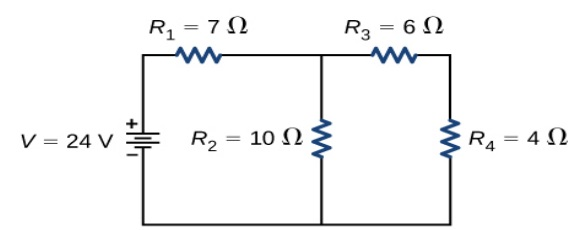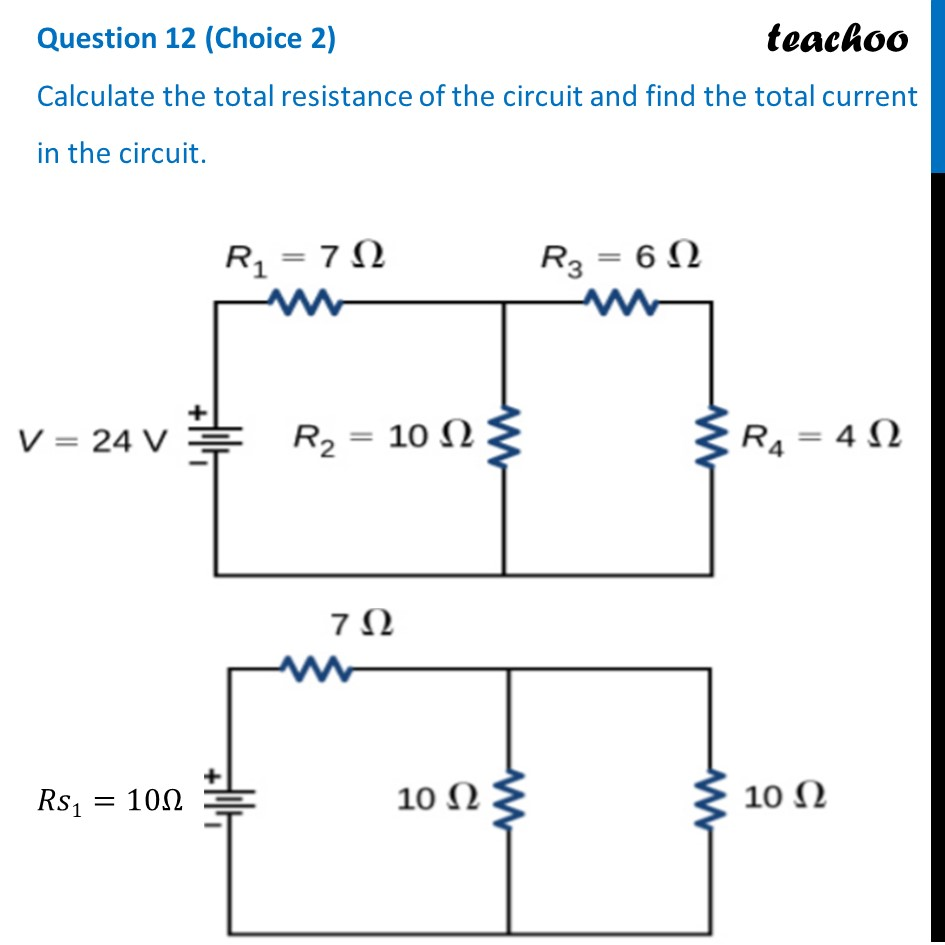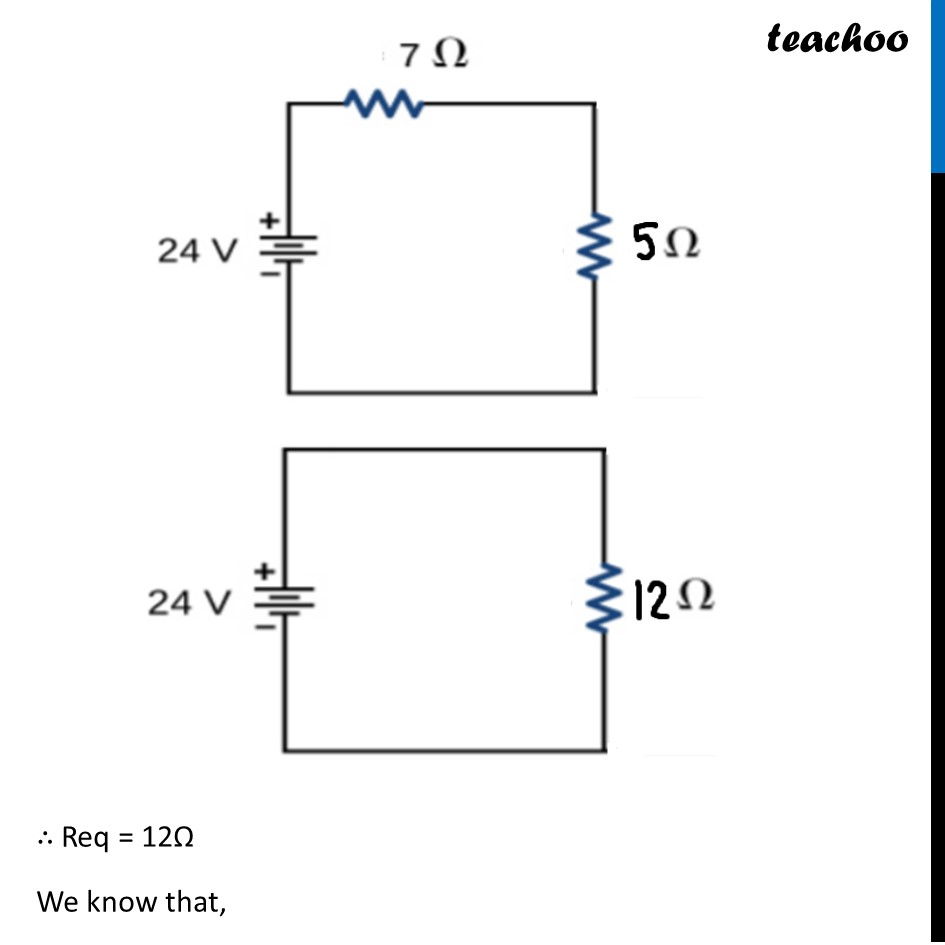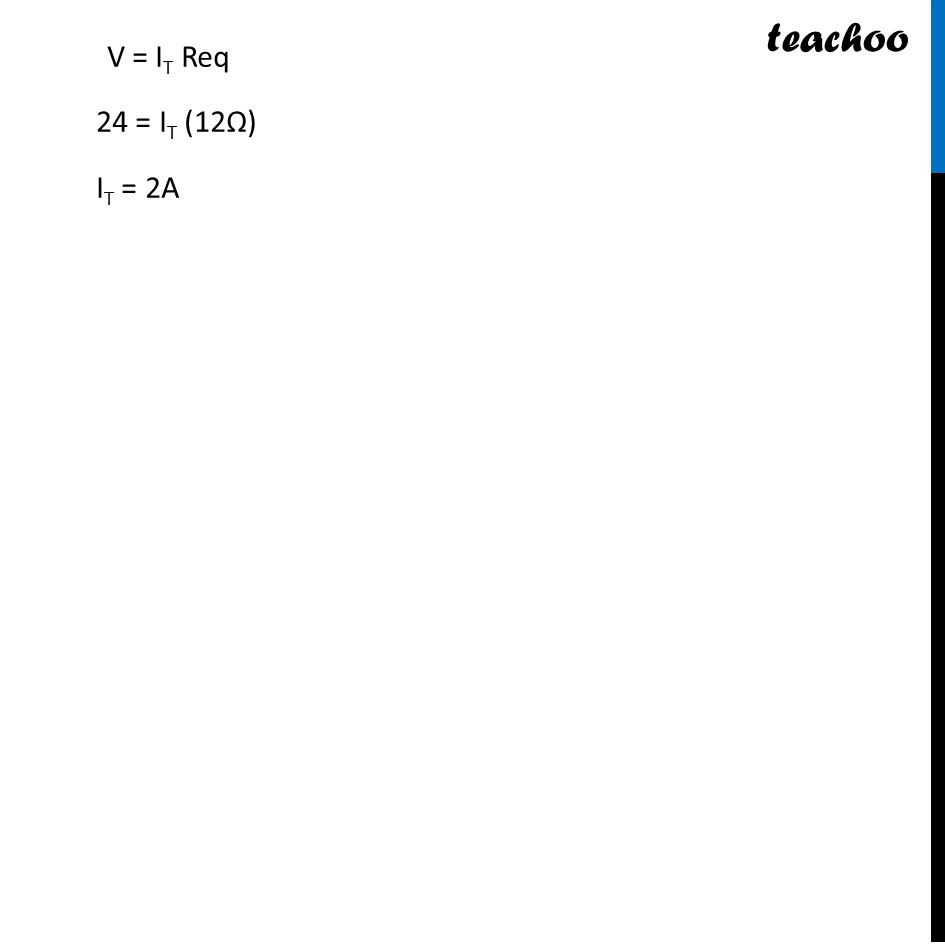CBSE Class 10 Sample Paper for 2022 Boards [Term 2] - Science Solution

Class 10
Solutions to CBSE Sample Paper - Science Class 10## Calculate the total resistance of the circuit and find the total current in the circuit.Learn in your speed, with individual attention - Teachoo Maths 1-on-1 Class

### Transcript

Question 12 (Choice 2) Calculate the total resistance of the circuit and find the total current in the circuit. ∴ Req = 12Ω We know that, V = IT Req 24 = IT (12Ω) IT = 2A